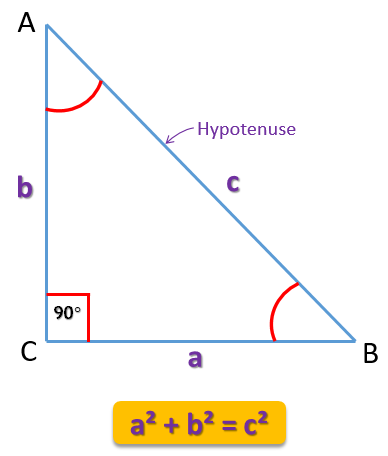Pythagorean Triples

As per Pythagoras Theorem, we get expressions for right angled $\triangle ABC$;

$a^2 + b^2 = c^2$Pythagoras Theorem

By plotting some values for 'a' and 'b', one may get value of 'c' or in other words,

One may find value of Hypotenuse (longest side) by using values of other two sides.

So, if we have, $a=3 \ and \ b=4$, then by  Pythagoras Theorem,

\begin {aligned} c^2 &= 3^2 + 4^2 \\ \therefore c^2 &= 9 + 16 \\ \therefore c^2 &= 25 \\ \therefore c &= \sqrt {25} \\ \therefore c &= 5\end {aligned}

So, we get (3, 4, 5) as one of the Pythagorean triple.

Meaning, the square of the largest number will be the sum of the squares of the other two.

You can find popular triplets as,

(3, 4 ,5)
(6, 8, 10)
(12, 13, 5)

You can check the triples above by calculating yourself. Also, try to make some Pythagorean triplets by yourself.